# Current Electricity Physics What is Current Electricity Electricity

• Slides: 43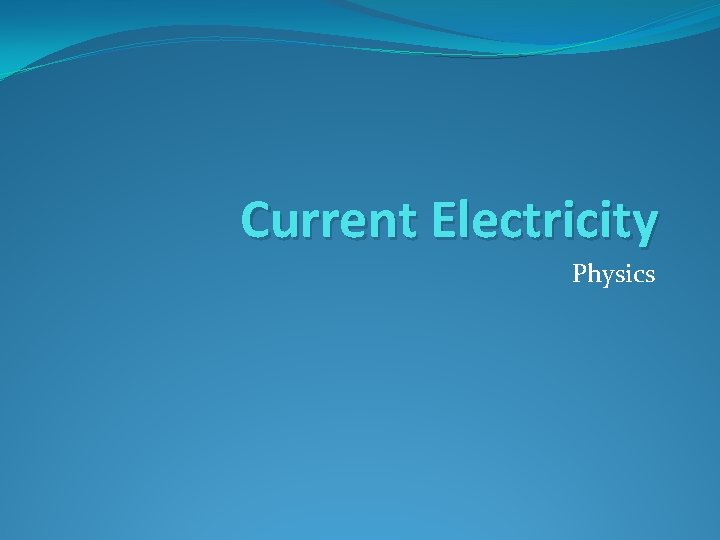Current Electricity Physics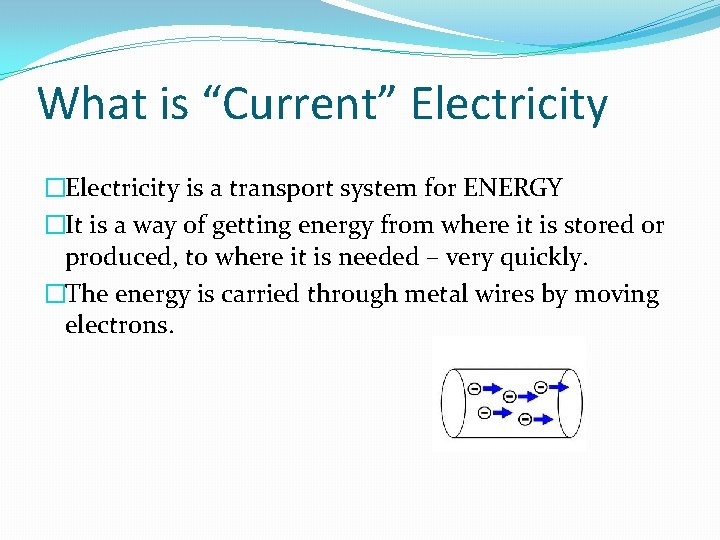What is “Current” Electricity �Electricity is a transport system for ENERGY �It is a way of getting energy from where it is stored or produced, to where it is needed – very quickly. �The energy is carried through metal wires by moving electrons.Electric Current �An electric current is a flow of negative charge (electrons) �Charge is measured in coulombs. (There actually 62500000000 electrons in 1 coulomb) �The number of coulombs flowing past a point in 1 second is called the current. �Current is measured in amps (A) � 3 A means 3 coulombs per second �watchCharge Current and time �The total amount of charge flowing can be calculated using: Q is the charge in coulombs (C �Q = I x t ) I is the current in amps (A) t is the time in seconds (s) Example : a current of 3 A flows through a bulb for 20 minutes. What is the total amount of charge that has flowed through the bulb? Q = I x t = 3 x (20 x 60) = 3600 CMeasuring Current �Current is measured with an ammeter. �The ammeter is connected in- line with the circuit. �Current is measured in amps. �The current is the same at all points around a circuit which only has one path.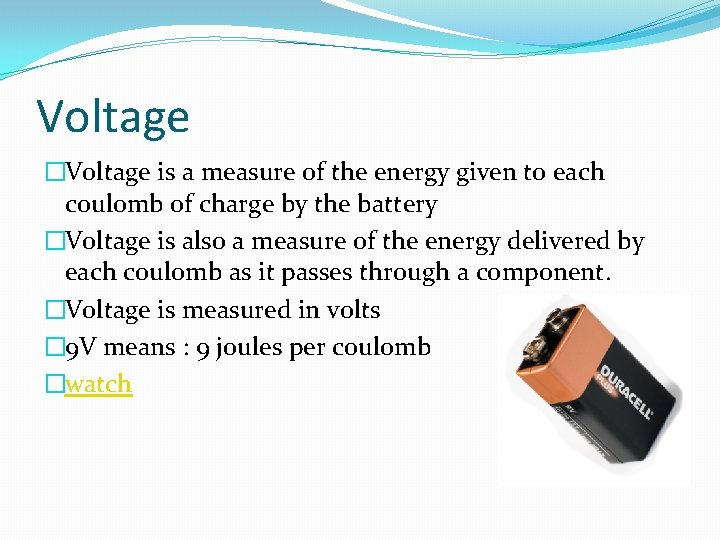Voltage �Voltage is a measure of the energy given to each coulomb of charge by the battery �Voltage is also a measure of the energy delivered by each coulomb as it passes through a component. �Voltage is measured in volts � 9 V means : 9 joules per coulomb �watch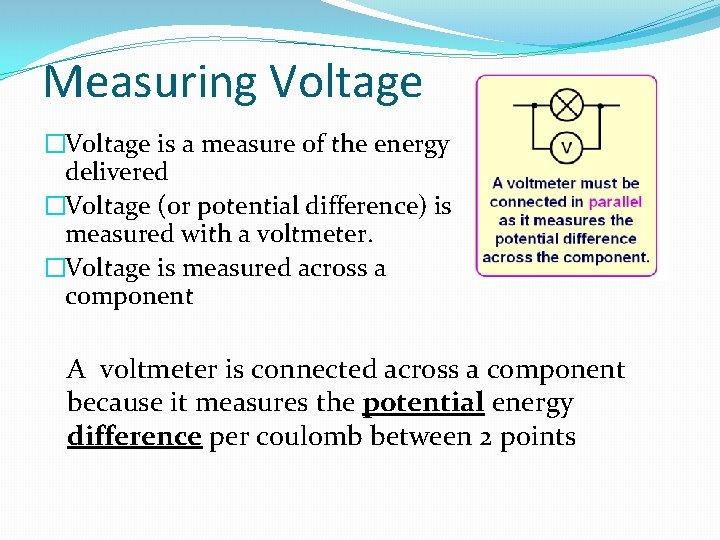Measuring Voltage �Voltage is a measure of the energy delivered �Voltage (or potential difference) is measured with a voltmeter. �Voltage is measured across a component A voltmeter is connected across a component because it measures the potential energy difference per coulomb between 2 points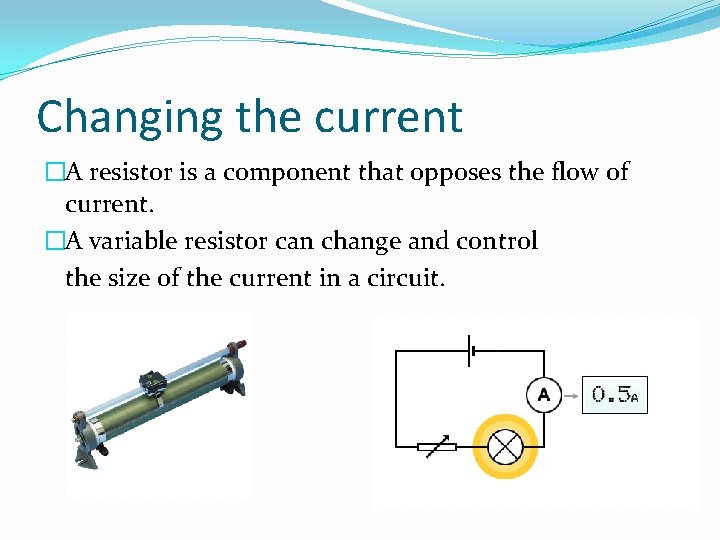Changing the current �A resistor is a component that opposes the flow of current. �A variable resistor can change and control the size of the current in a circuit.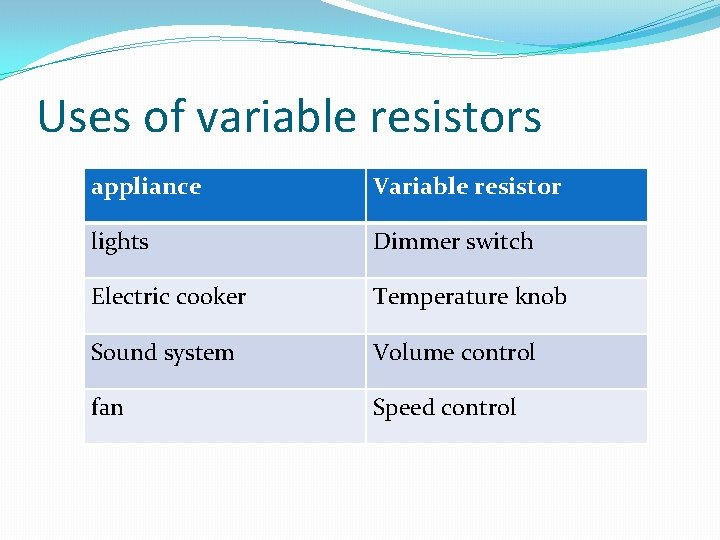Uses of variable resistors appliance Variable resistor lights Dimmer switch Electric cooker Temperature knob Sound system Volume control fan Speed controlInvestigating Resistance Starting with one cell measure voltage across the resistor and the current through it. Repeat for 2 , 3 and 4 cells. Plot a graph of your results.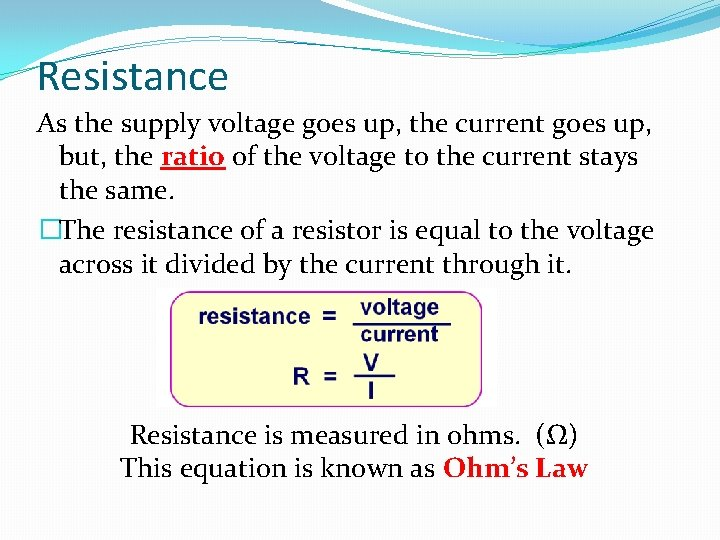Resistance As the supply voltage goes up, the current goes up, but, the ratio of the voltage to the current stays the same. �The resistance of a resistor is equal to the voltage across it divided by the current through it. Resistance is measured in ohms. (Ω) This equation is known as Ohm’s LawOhm’s Law graph Current (m. A) 1. 5 3. 0 4. 5 6. 0 32 64 96 128 140 120 Current (m. A) Voltage (V) Ohm's Law experiment 100 80 60 40 20 0 0. 0 2. 0 4. 0 Voltage (V) 6. 0 The graph is a straight line through the origin because the voltage divided by the current is a constant The resistance is constant (in this case 47 Ω) 8. 0The strange case of the light bulb When an old fashioned light bulb is turned on, as it starts to glow it heats up! As it heats up the resistance of its filament increases. The means that the graph of current and voltage is not a straight line through the origin. This is because the resistance of the bulb is not constant. (Thank goodness we use LEDs now)Ohms law example The meter readings are: Voltmeter = 12 V Ammeter = 3 A Calculate the resistance of the resistor.Series Circuits - currentSeries Circuit - voltage • In a series circuit the bulbs are in the same path so they share the battery voltage. • The voltage across each bulb adds up to the battery voltage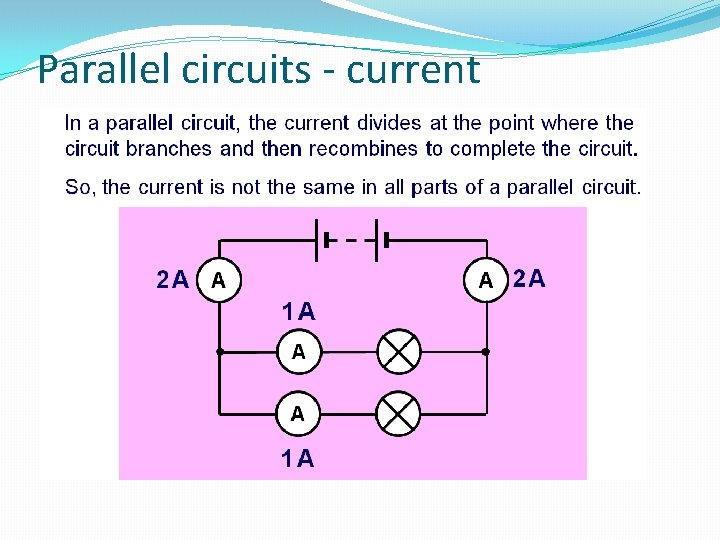Parallel circuits - currentParallel circuit - voltage • In a parallel circuit each bulb is directly connected to the battery in its own path. • Each bulb gets the full battery voltage. • The voltage across each bulb is the same as the battery voltage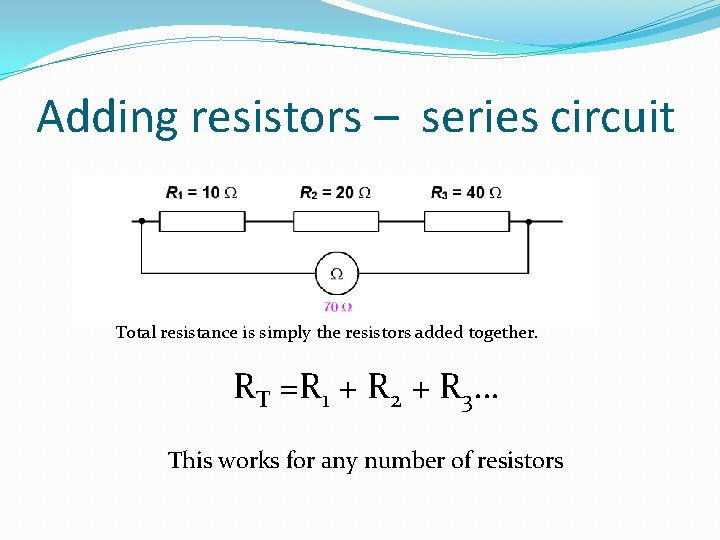Adding resistors – series circuit Total resistance is simply the resistors added together. RT =R 1 + R 2 + R 3… This works for any number of resistorsAdding resistors in parallel The total resistance of resistors in parallel is always smaller than the smallest resistor. We need a special equation to work out the total.Adding Resistors in Parallel �There is a great video on how to use a Casio fx 83 calculator to work out the total resistance of resistors in parallel…. �On mrstewartphysics on You. Tube Click Here. .Power �Power is a measure of the energy used per second. �Energy is measured in joules (J) �Power is measured in watts (W) � 100 watts is equal to 100 joules used per second.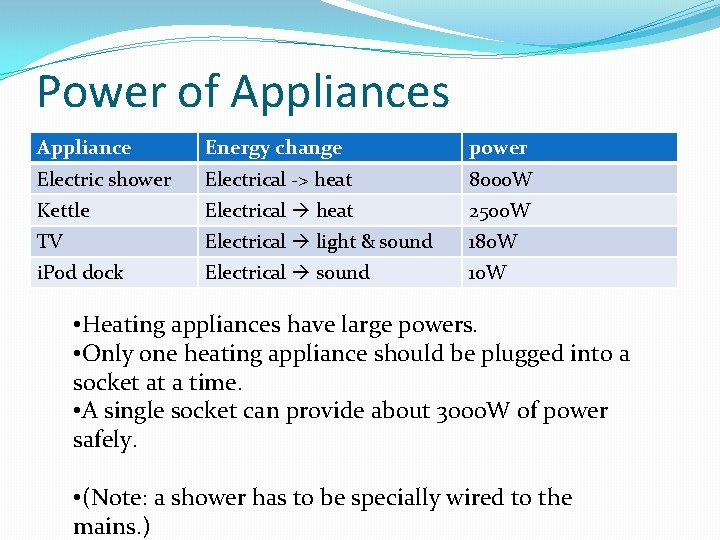Power of Appliances Appliance Energy change power Electric shower Electrical -> heat 8000 W Kettle Electrical heat 2500 W TV Electrical light & sound 180 W i. Pod dock Electrical sound 10 W • Heating appliances have large powers. • Only one heating appliance should be plugged into a socket at a time. • A single socket can provide about 3000 W of power safely. • (Note: a shower has to be specially wired to the mains. )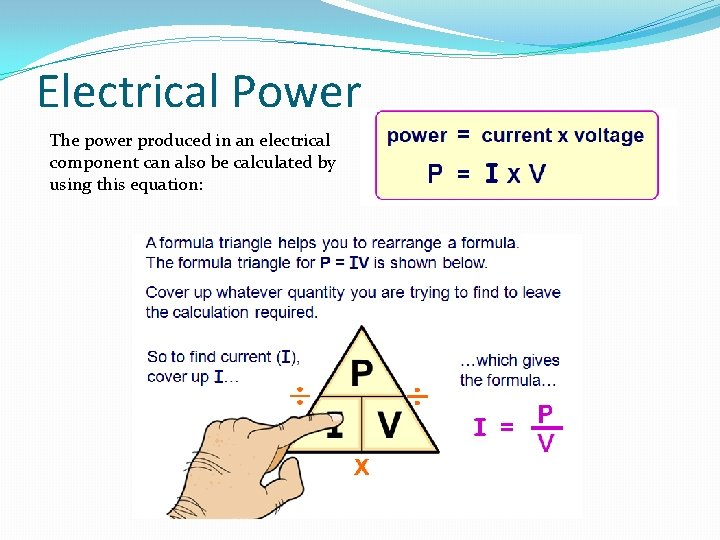Electrical Power The power produced in an electrical component can also be calculated by using this equation: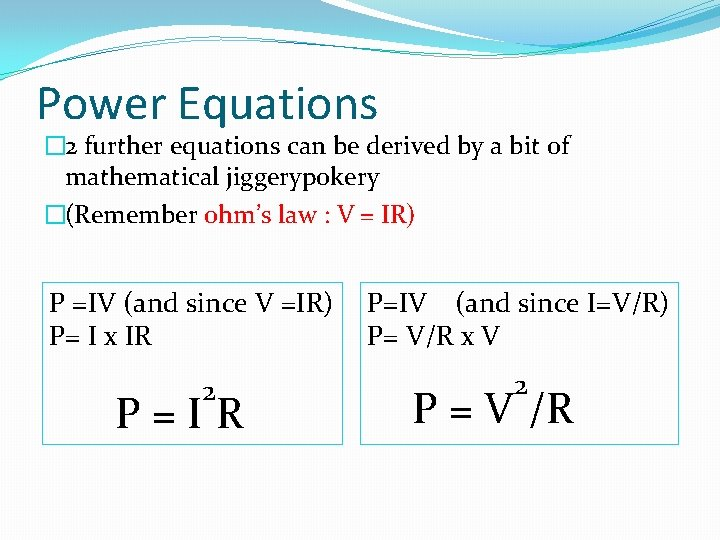Power Equations � 2 further equations can be derived by a bit of mathematical jiggerypokery �(Remember ohm’s law : V = IR) P =IV (and since V =IR) P= I x IR 2 P = I R P=IV (and since I=V/R) P= V/R x V P = V 2 /R4 Power equations �So we now have 4 electrical power equations But which one do we use? . . . . Well, it depends on the question…………………. .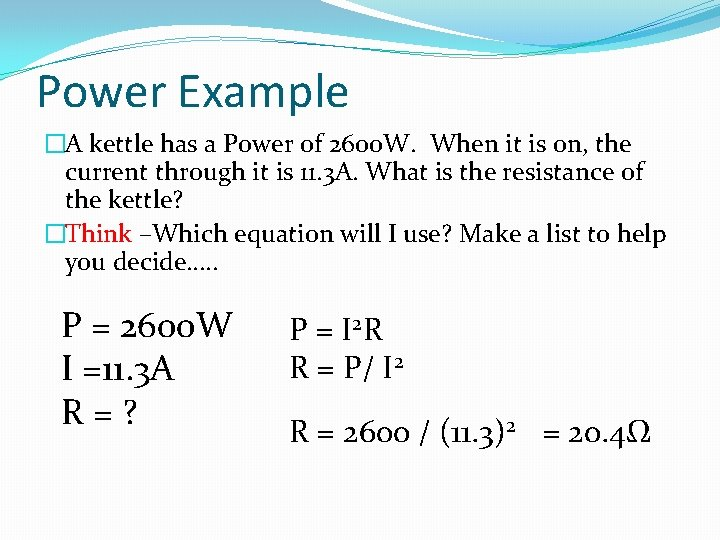Power Example �A kettle has a Power of 2600 W. When it is on, the current through it is 11. 3 A. What is the resistance of the kettle? �Think –Which equation will I use? Make a list to help you decide…. . P = 2600 W I =11. 3 A R = ? P = I 2 R R = P/ I 2 R = 2600 / (11. 3)2 = 20. 4ΩDC electricity (Direct Current) �The electrical energy we get from batteries and solar cells is transferred from where it is stored or produced to where it is required by a flow of charge in one direction �We say that current is a flow of negative charge (electrons) that flows from negative to positive.AC electricity (Alternating Current) �AC electricity is produced by a “generator”. This is a spinning magnet inside a stationary coil of wire. �The electricity flows one way then the other. The frequency of this alternating current depends on the frequency of rotation of the generator. �In the UK our electricity is produced using generators that spin 50 times a second (50 Hz) �This alternating current is pushed one way and than pulled back the other way. This means that the flow of charge is always changing!Analogy – Sawing a piece of wood There are 2 ways to cut a piece of wood in half, both do the job, both transfer energy. Circular saw – one direction Saw moves at a steady speed in one direction (DC) Handsaw – changes direction Saw changes speed and changes direction (AC)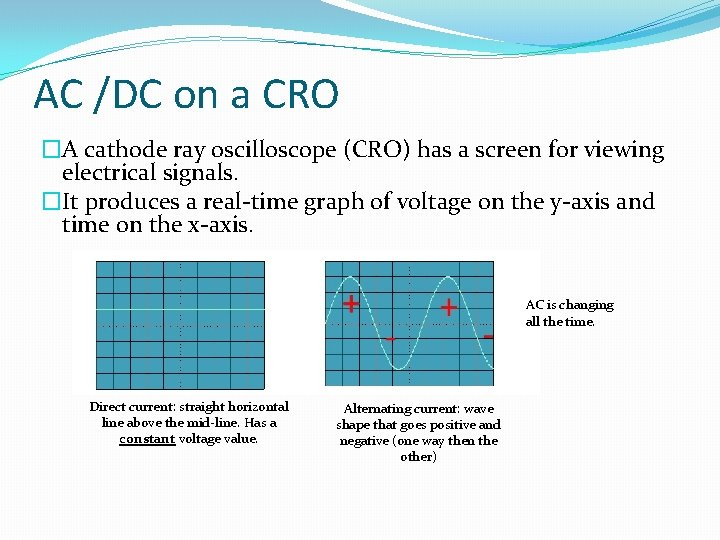AC /DC on a CRO �A cathode ray oscilloscope (CRO) has a screen for viewing electrical signals. �It produces a real-time graph of voltage on the y-axis and time on the x-axis. AC is changing all the time. Direct current: straight horizontal line above the mid-line. Has a constant voltage value. Alternating current: wave shape that goes positive and negative (one way then the other)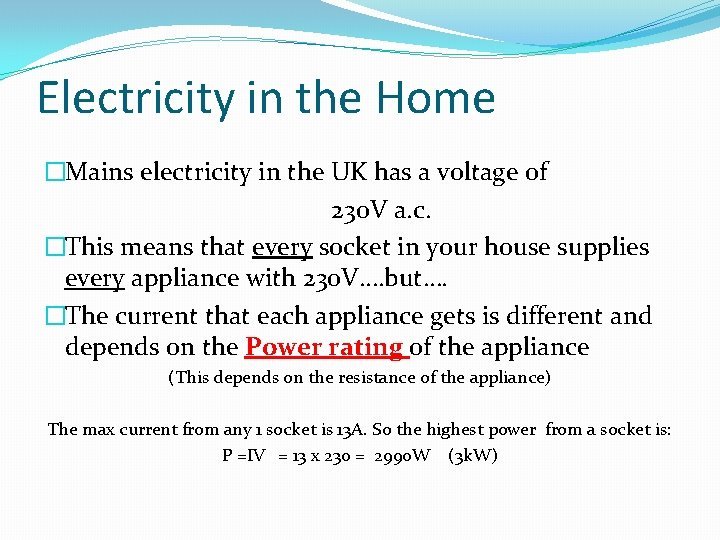Electricity in the Home �Mains electricity in the UK has a voltage of 230 V a. c. �This means that every socket in your house supplies every appliance with 230 V…. but…. �The current that each appliance gets is different and depends on the Power rating of the appliance (This depends on the resistance of the appliance) The max current from any 1 socket is 13 A. So the highest power from a socket is: P =IV = 13 x 230 = 2990 W (3 k. W)The Plug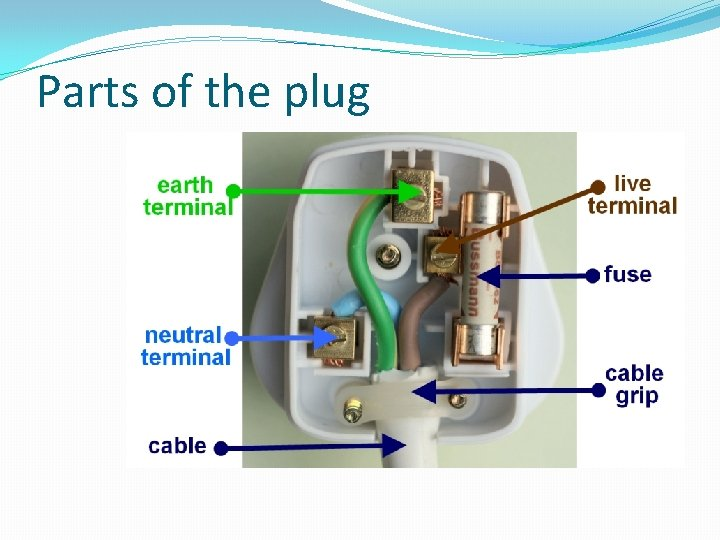Parts of the plug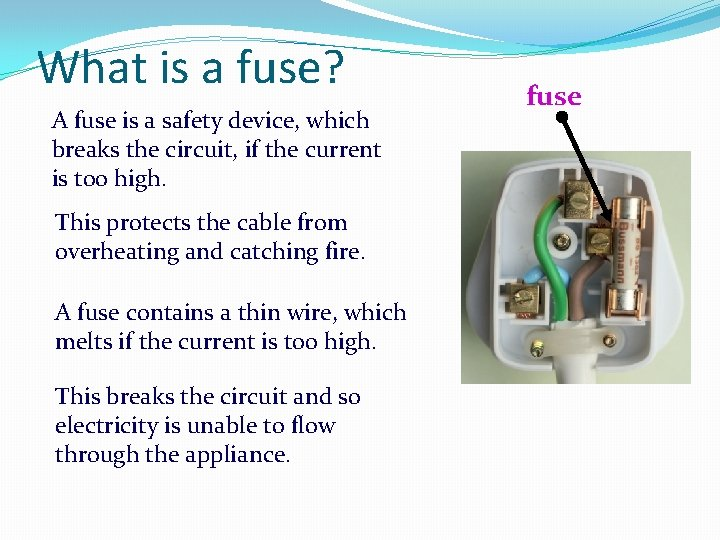What is a fuse? A fuse is a safety device, which breaks the circuit, if the current is too high. This protects the cable from overheating and catching fire. A fuse contains a thin wire, which melts if the current is too high. This breaks the circuit and so electricity is unable to flow through the appliance. fuse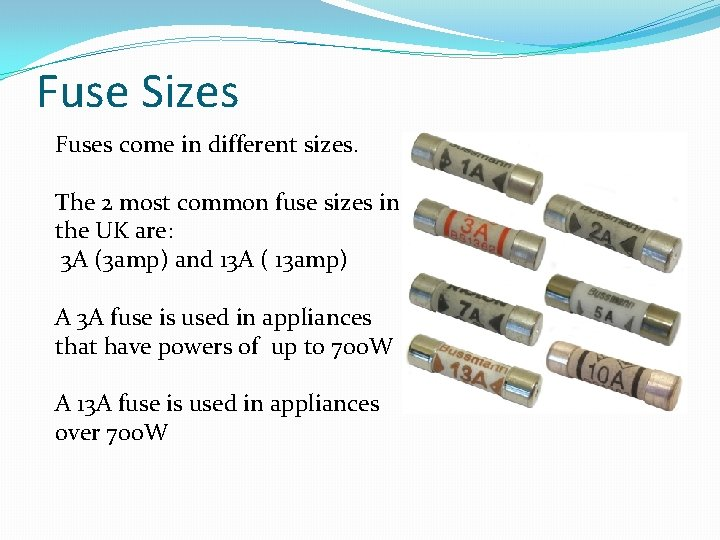Fuse Sizes Fuses come in different sizes. The 2 most common fuse sizes in the UK are: 3 A (3 amp) and 13 A ( 13 amp) A 3 A fuse is used in appliances that have powers of up to 700 W A 13 A fuse is used in appliances over 700 W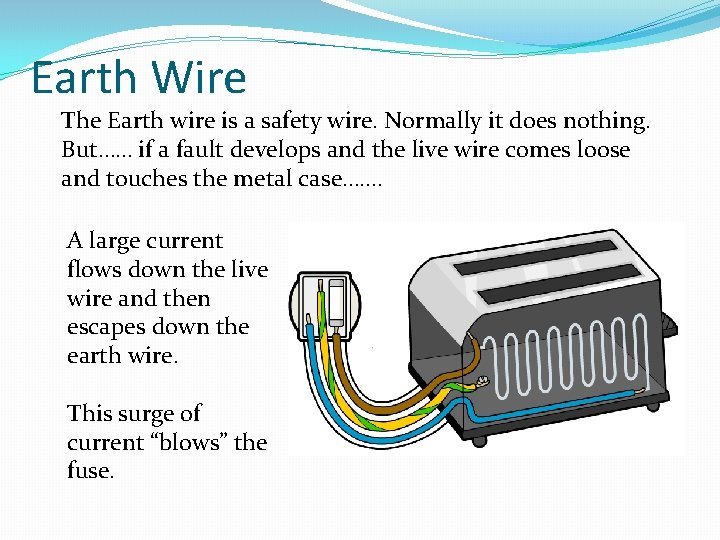Earth Wire The Earth wire is a safety wire. Normally it does nothing. But…… if a fault develops and the live wire comes loose and touches the metal case……. A large current flows down the live wire and then escapes down the earth wire. This surge of current “blows” the fuse.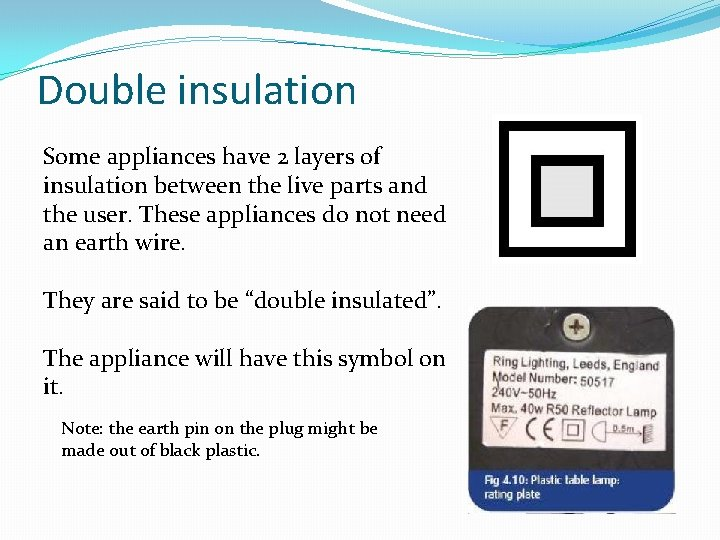Double insulation Some appliances have 2 layers of insulation between the live parts and the user. These appliances do not need an earth wire. They are said to be “double insulated”. The appliance will have this symbol on it. Note: the earth pin on the plug might be made out of black plastic.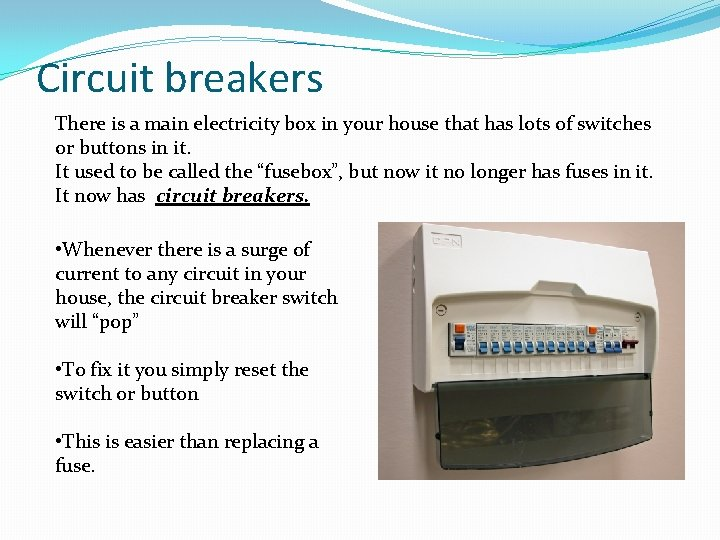Circuit breakers There is a main electricity box in your house that has lots of switches or buttons in it. It used to be called the “fusebox”, but now it no longer has fuses in it. It now has circuit breakers. • Whenever there is a surge of current to any circuit in your house, the circuit breaker switch will “pop” • To fix it you simply reset the switch or button • This is easier than replacing a fuse.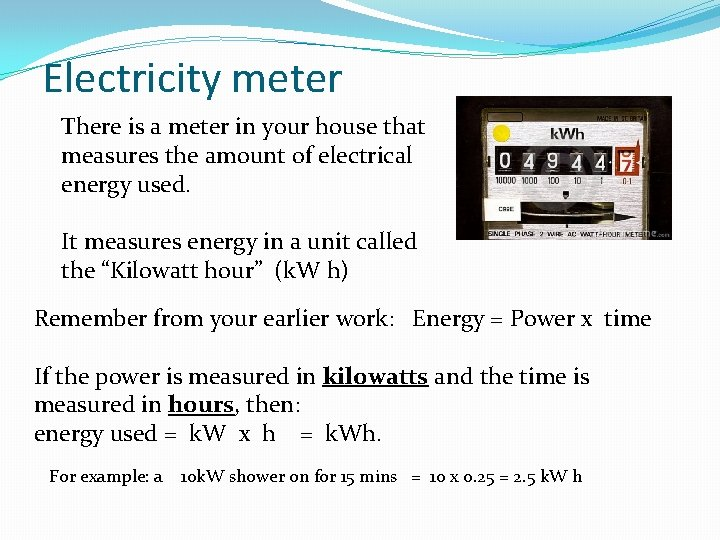Electricity meter There is a meter in your house that measures the amount of electrical energy used. It measures energy in a unit called the “Kilowatt hour” (k. W h) Remember from your earlier work: Energy = Power x time If the power is measured in kilowatts and the time is measured in hours, then: energy used = k. W x h = k. Wh. For example: a 10 k. W shower on for 15 mins = 10 x 0. 25 = 2. 5 k. W hThe cost of electricity Electricity companies charge customers per “kilowatt hour” k. Wh In 2019 the approximate cost of a “kilowatt hour” is 12 pence.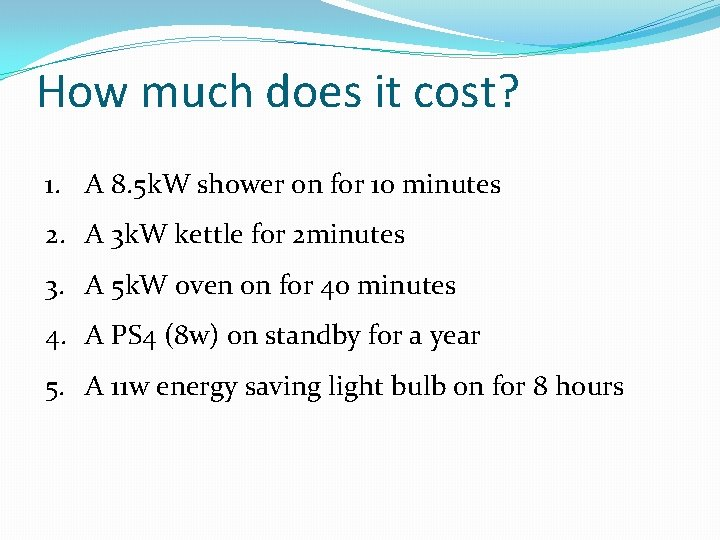How much does it cost? 1. A 8. 5 k. W shower on for 10 minutes 2. A 3 k. W kettle for 2 minutes 3. A 5 k. W oven on for 40 minutes 4. A PS 4 (8 w) on standby for a year 5. A 11 w energy saving light bulb on for 8 hours